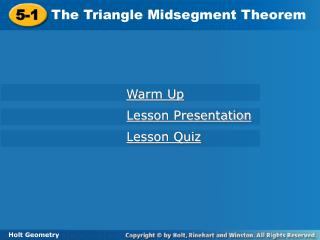DownloadDownload Presentation5-1

# 5-1

Télécharger la présentation## 5-1

- - - - - - - - - - - - - - - - - - - - - - - - - - - E N D - - - - - - - - - - - - - - - - - - - - - - - - - - -
##### Presentation Transcript

1. 5-1 The Triangle Midsegment Theorem Warm Up Lesson Presentation Lesson Quiz Holt Geometry

2. Warm Up Use the points A(2, 2), B(12, 2) and C(4, 8) for Exercises 1–5. 1.Find X and Y, the midpoints of AC and CB. 2. Find XY. 3. Find AB. 4. Find the slope of AB. 5. Find the slope of XY. 6. What is the slope of a line parallel to 3x + 2y = 12? (3, 5), (8, 5) 5 10 0 0

3. Objective Prove and use properties of triangle midsegments.

4. Vocabulary midsegment of a triangle

5. A midsegment of a triangleis a segment that joins the midpoints of two sides of the triangle. Every triangle has three midsegments, which form the midsegment triangle.

6. The vertices of ∆XYZ are X(–1, 8), Y(9, 2), and Z(3, –4). M and N are the midpoints of XZ and YZ. Show that and . Example 1: Examining Midsegments in the Coordinate Plane Step 1 Find the coordinates of M and N.

7. Step 2 Compare the slopes of MN and XY. Since the slopes are the same, Example 1 Continued

8. Step 3 Compare the heights of MN and XY. Example 1 Continued

9. The relationship shown in Example 1 is true for the three midsegments of every triangle.

10. Example 2A: Using the Triangle Midsegment Theorem Find each measure. BD ∆ Midsegment Thm. Substitute 17 for AE. BD = 8.5 Simplify.

11. Example 2B: Using the Triangle Midsegment Theorem Find each measure. mCBD ∆ Midsegment Thm. mCBD = mBDF Alt. Int. s Thm. mCBD = 26° Substitute 26° for mBDF.

12. Lesson Quiz: Part I Use the diagram for Items 1–3. Find each measure. 1.ED 2.AB 3. mBFE 10 14 44°

13. Lesson Quiz: Part II 4. Find the value of n. 5.∆XYZ is the midsegment triangle of∆WUV. What is the perimeter of∆XYZ? 16 11.5